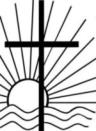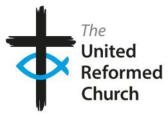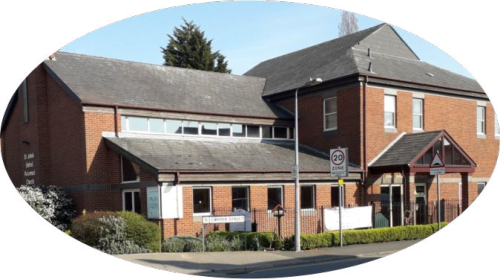### h

1 C o w p e r S t r e e t , I p s w i c h I P 4 5 J D W e b   A d d r e s s : k

## r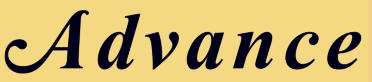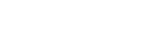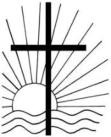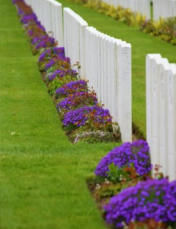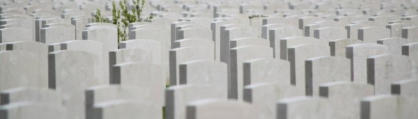2

### Web:

Te l :0 1 4 7 3 7 2 4 9 6 1 ( C u r r e n t l y not avail able) k k M i n i s t e r s L ett e r R e m e m b e r i n g
A f e w y e a r s a g o , a s p a r t o f a s t u d y t o u r ( E a s t e r n S y n o d M i n i s t e r s S p r i n g S c h o o l ) , u s m i n i s t e r s s p e n t 5 d a y s i n F r a n c e a n d B e l g i u m . ( N B S o m e w h a t b i z a r r e l y s p e n d i n a w e e k i n n o r t h e r n F r a n c e i n c l u d i n g c o a c h a n d t u n n e l a n d a c c o m m o d a t i o n i s s i g n i f i c a n t l y c h e a p e r t h a n a n y w h e r e i n U K ! ) . T h e t h e m e w a s t i t l e d I n F l a n d e r s F i e l d , t h r o u g h m u d a n d b l o o d t o g r e e n f i e l d s b e y o n d s i t e s o f p e a c e a n d r e c o n c i l i a t i o n o n t h e w e s t e r n f r o n t . I l i k e s h o r t s n a p p y t i t l e s ! O v e r t h e w e e k w e v i s i t e d s i g n i f i c a n t W W 1 s i t e s i n n o r t h e r n F r a n c e a n d W e s t e r n B e l g i u m . W e r e f l e c t e d o n t h e i s s u e o f w a r a n d p e a c e b o t h i n t h e p a s t a n d t h e i s s u e s t h a t a r i s e i n t h e p r e s e n t d a y . O u r f a c i l i t a t o r s w e r e t w o M e n n o n i t e s , w h o a r e p a c i f i s t s , a n d a f o r m e r A i r F o r c e c h a p l a i n w h o i s a U R C m i n i s t e r . T h e l e c t u r e s a n d d i s c u s s i o n w h e r e s u p p o r t e d b y e x c u r s i o n s t o s i t e s i n a n d a r o u n d Y p r e s i n B e l g i u m . O f t h e m a n y i m p r e s s i o n s a n d e n c o u n t e r s d u r i n g t h e w e e k , I t h i n k t h e o n e t h a t m a d e m o s t i m p r e s s i o n o n m e w a s t h e v i s i t t o T y n e C o t C e m e t e r y . T h o u g h t h e r e a r e a b o u t a 1 0 0 B r i t i s h a n d C o m m o n w e a l t h m i l i t a r y c e m e t e r i e s i n t h e a r e a , T y n e C o t i s t h e l a r g e s t . I n f a c t i t i s t h e l a r g e s t W W 1 c o m m o n w e a l t h c e m e t e r y i n t h e w o r l d . A l m o s t 1 2 , 0 0 0 s e r v i c e m e n a r e b u r i e d t h e r e , 8 0 0 0 u n i d e n t i f i e d . A r o u n d t h e e d g e i s a w a l l w i t h 3 5 , 0 0 0 n a m e s o f s o l d i e r s w h o s e b o d i e s w e r e n e v e r r e c o v e r e d . T h e s e a l l f e l l d e f e n d i n g Y p r e s 1 9 1 4 - 1 8 , a n d t h e i r n a m e s c o u l d n t f i t o n t h e M e n i m G a t e i n Y p r e s . T h e r o w o n r o w o f t h o u s a n d s o f w h i t e g r a v e s t o n e s w a s i n c r e d i b l y m o v i n g . T h e c e m e t e r y w a s b o t h b e a u t i f u l a n d h o r r i f i c a l l a t t h e s a m e t i m e . I t w a s b e a u t i f u l b e c a u s e t h e m a n i c u r e d l a w n s , f l o w e r s , h e a d s t o n e s a n d m e m o r i a l s h a v e a p i c t u r e s q u e a p p e a l . I t w a s h o r r i f i c a s b e h i n d e v e r y h e a d s t o n e a n d n a m e l a y a p e r s o n w h o s e G o d g i v e n p o t e n t i a l w a s c u t o f f , f r e q u e n t l y a t a n e x c e p t i o n a l l y y o u n g a g e . I t w a s m o v i n g , i t w a s s a d d e n i n g , i t r e m i n d e d m e a g a i n o f w h y w e c e l e b r a t e R e m e m b r a n c e D a y . I t w a s i n t e r e s t i n g i n t h e d i s c u s s i o n b o t h i n f o r m a l a n d g u i d e d , t h a t w e a l l a g r e e d t h a t w a r i s w r o n g , t h a t i t i s e v i l a n d c a u s e s e v i l . W h e r e t h e d i s c u s s i o n b e c a m e i m p a s s i o n e d w a s o v e r t h e i s s u e o f w h e t h e r w a r c a n b e u s e d t o s t o p e v e n g r e a t e r e v i l , a n d i f t h a t i s t h e c a s e i f i t c a n e v e r b e c a l l e d j u s t . W e l i v e i n a m e s s y , f a l l e n w o r l d , a n d t h o u g h w e w o u l d l i k e t o t h i n k s o , t h e r e i s n o e a s y a n s w e r . J e s u s d i d s a y , h o w e v e r B l e s s e d b e t h e p e a c e m a k e r s . A n d w h e t h e r w e a r e p a c i f i s t s o r b e l i e v e t h a t s o m e t i m e s w a r i s a n e c e s s a r y e v i l , w e n e e d t o s e e k a t r u e a n d j u s t p e a c e b e t w e e n n e i g h b o u r a n d n a t i o n . A n d m a k i n g a n d s u s t a i n i n g p e a c e i s f r e q u e n t l y h a r d e r w o r k t h a n a n g e r , g u n s o r w a r . I n t h i s u n c e r t a i n w o r l d , I p r a y t h a t w e c a n d o t h e h a r d w o r k o f p e a c e - m a k i n g a n d i f w e d o , G o d w i l l b l e s s u s . David
Photos including co ver ta k en by Rev David R e e s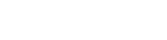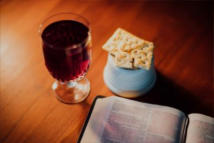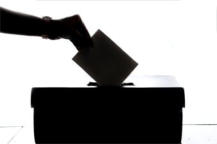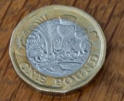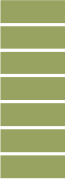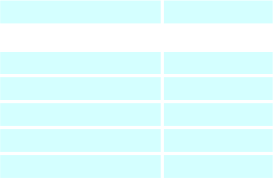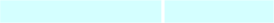3
C H R I S T I A N I T Y E X P L O R E D
Chur ch Secr e t ar y s Not es These ar e dif ficult times, but it s g ood t o he a r tha t some of the gr oup s tha t use our bui lding ha v e been in t ouch t o le t me kno w ho w the y ar e g e t ting on. The AA a r e looking f or w ar d t o when the y c an mee t ag ai n in our building and the y ar e mee ting on line but tha t is no t as bene f icial. The y send their r eg ar ds. The Br o wnies ar e ‘Z ooming ’ t oo and the Pr eschool ar e w ell or g anised and enjo ying mee ting , although with f e w er childr en. Member s of Impr o visa tions ha v e been making mask s and another gr oup has been t aking i t i n turns m ee ting e v er y Sa tur da y morning armed with glo v es and s a n i t i z e r s p r a y t o mak e sur e the chur ch is sa f e f or Sunda y s er vices. Gr ea t. W ell done t o y ou a ll! If y ou w oul d lik e t o help in an y w a y , le t me kno w . The Elder s held an e x tr a mee ting t o c onsider if w e c ould sa f ely hold a c ommunion ser vice a t St Johns. Aft er a though tful discussion, with all elder s e xpr essing their vie w , it w as decided, sadly , tha t in the li gh t of the w or sening virus situa tion, w e should not hol d a c ommunion ser vice in the chur ch f or the r es t of the y ear . W e wil l r e v i s i t t h is t o pic early ne x t y ear . Elder s elections ar e due t o t ak e place soon. John, K a thleen, P e t er and Andr e w ha v e all c ome t o the end of their period in of f ice and ar e due t o s t and do wn. This means w e need nomina tions f or r epla c emen ts. Nomina tion f o rm s ar e a v ailabl e i n the chur ch or Adv ance (see las t pag e) and need t o be giv en t o me b y 15th No v ember . Please mak e sur e the per son y ou nomina t e is happ y t o s t and! The se ar e tricky times f or elections so y o u c an alw a y s email or phone me with nomina tions if y ou c an’t g e t t o Chur ch. K eep Sa f e. Adrian T h e s t a r t d a t e h a s b e e n m o v e d t o W e d n e s d a y e v e n i n g 1 3 t h J a n u a r y v i a Z o o m . C a n I n e v e r t h e l e s s a s k y o u t o c o n t i n u e t o p r a y f o r t h i s
i n i t i a t i v e . I t i s v i t a l t h a t w e a l l o w p e o p l e o n t h e f r i n g e a n d t h o s e w i t h q u e s t i o n s t o e x p l o r e a n d h o p e f u l l y d i s c o v e r t h e m e a n i n g o f l i f e a s r e v e a l e d b y J e s u s . A l s o i f t h e r e a r e f r i e n d s , r e l a t i v e s , n e i g h b o u r s o r c o n t a c t s t h a t y o u f e e l w o u l d w e l c o m e a n i n v i t a t i o n , p l e a s e l e t o n e o f t h e m i n i s t r y t e a m k n o w . D a v i d
T r e a s u r e r s R e p o r t Y o u a r e t h a n k e d f o r y o u r O f f e r i n g s : S e p t e m b e r £ 1 , 6 6 8 . 0 0 A u g u s t £ 1 , 7 4 3 . 5 0 . Y o u w i l l h a v e r e a d l a s t m o n t h t h a t A n g i e a n d B r i a n S a v a g e , h a v i n g r e t u r n e d t o t h e U . K . , w i l l n o t b e r e t u r n i n g t o c o n t i n u e t h e i r w o r k i n T a n z a n i a . W e a r e g r a t e f u l t o a l l t h o s e w h o h a v e m a d e d o n a t i o n s / k n i t t e d t o y s e t c t o a s s i s t i n t h e i r w o r k o v e r m a n y y e a r s , w e k n o w t h e y h a v e b e e n v e r y a p p r e c i a t i v e o f S t J o h n s h e l p o v e r t h i s l e n g t h y p e r i o d . E l d e r s w i l l b e d e c i d i n g o n t h e d e s t i n a t i o n o f t h i s y e a r ' s C h r i s t m a s d o n a t i o n s i n d u e c o u r s e . O u r A n n u a l G i f t D a y t h i s y e a r i s o n 1 s t N o v e m b e r W e w i l l n o t b e d i s t r i b u t i n g t h e u s u a l e n v e l o p e s a s i n p r e v i o u s y e a r s , a s d u e t o c u r r e n t s i t u a t i o n , w o u l d p r e s e n t t o o m a n y d i f f i c u l t i e s f o r u s . W e w o u l d b e g r a t e f u l , t h e r e f o r e , f o r a l l d o n a t i o n s t o b e b y b a n k t r a n s f e r o r c h e q u e ( p r e f e r a b l y n o t c a s h ) , g i f t a i d e d w h e r e y o u c a n . I f d o n a t i n g b y c h e q u e - p l e a s e e n s u r e p a y e e i s s t a t e d i n f u l l : S T J O H N S U N I T E D R E F O R M E D C H U R C H t h i s i s a r e q u i r e m e n t o f o u r B a n k e r s - - T h a n k s . T h e y c a n b e s e n t t o e i t h e r o f u s o r t o t h e C h u r c h . S t J o h n s i s e x t r e m e l y g r a t e f u l f o r y o u r c o n t i n u e d f i n a n c i a l s u p p o r t , p a r t i c u l a r l y i n t h e s e d i f f i c u l t d a y s M a n y t h a n k s M a r y A a n d R i c h a r d W Ser vice s in N o v ember 20 20Da t e
Online
01/11 Ernie *C 08/11 Da vid *R 15/11 Adam
22/11
Ernie
29/11 Da vid 06/12 Adam *C Ernie/Da vid *C = Communion *R = R emem br ance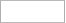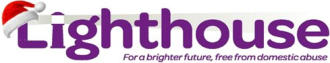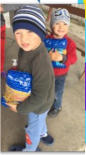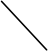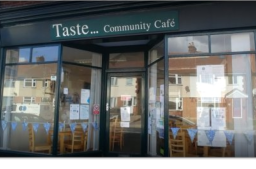4
Helping Other s
C h r i s t m a s G i f t C o l le c t i o n I d ea s 2 0 2 0 Lighthouse have agreed with St John’ s for us t o t a k e t o y s e t c., fr om our T o y Ser vice on 13 December t o them but y ou c an m a k e y o ur o wn arr ang emen ts, lik e g e t ting someone t o bring them t o the ser vice or sending them y our self . Belo w i s w ha t the y ha v e ask ed f or . Please c an all gifts be dona t ed un wr apped. Gift Car ds in £5 domina tions ar e e x tr emely use ful, these c an be fr om an y loc a l s t o r e s uch as Primark, The W ork s, Ar g os, B&M St or e, Sports Dir ect and an y supermark e t. These ar e w onderful f or l as t m i nut e g ifts f or arriv als jus t be f or e and in be t w een Chris tmas and Ne w Y ear .
We are not able to do this

## omen

Sc ar v es & Glo v es, P erfume/body Spr a y s/De o dor an ts Lip s tick Hair Styler s i.e. Hair Dr y er s, Str aigh t ener s, Curling T ong s Hair brush, Handbag , Cr a fts i.e. Se wing , T apes tr y , P ain ti ng Nigh tw ear , Dr essing Go wns/Slipp er s , Choc ola t es/S w ee ts, Body Lotions/Body But t er , Nail P olish/ P amper/Manicur e Se ts, F ace Mask s, Diaries/Calendar s, Fleecy Thr o w s, Mak e Up or Mak e Up Se ts, D VDs (non violen t) Choc o la t es! W r apping paper , gift t ags and sellot ape ar e also e x tr emely use ful.

## For Children

Out door T o y s, E arl y Y ear s Shapes, St ack er s, Bab y T ee thing T o y s , Friction Engine T y pe, T o y sDolls/Barbie/ Action Figur es, Dr essing Up Clothes, Ba th T o y s , Happ y Land Pla y Se t s, Boar d Games, Car s & T ruck s, Leg o/Duplo , Electr onic Games, Cr a ft Se ts/Pla y Doug h, D VDs (under 15s), R oller sk a t es/Sc oot er s, Choc ola t e/S w ee ts, Dr essing Go wns/Slipper s/Nigh tw ear , F ootballs/Balls/Hoola Hoop s
Wha t ar e the bes t i t ems t o dona t e t o FIND in the run -up t o Chris tmas? T ub s of s w ee ts, choc ola t es and biscuits f or its Chris tmas hamper s; Biscuits, crisp s, nuts, t ea, c of f ee, sug ar , long lif e UHT milk, long lif e f ruit juice, cer eal; T oile tries such as soap, bo dy w ash, deodor an t. It ems the y don't need a t t he m o men t ar e: pas t a, soup, bak ed beans, porri dg e.
Our Har v es t dona tions this y ear w en t t o FIND , f or which the y w er e v er y gr a t e ful and send their thank s.  Lig h t ho us e w er e unable t o accep t f ood it ems a t this time but I am sur e w e will mak e dona tions t o them in the futur e. Mary Aldous
Open Monda y , W edn e sda y , Frida y & Sa tur da y 9 am t o 12 noon
Jus t t o le t y ou kno w w e ha v e placed the c ollection bo x es f or FIND jus t b y the door in t o the W or ship Ar ea. Ov er the las t eigh t mon ths FIND ha v e been v er y bus y supporting those in need and I kno w the y will appr e c ia t e us r es t arting our c ollections. If y o u ar e not able t o g e t t o chur ch a t the momen t  but w ou ld lik e t o c on tribut e, p lease giv e me a c all and I will be happ y t o c ollect fr om y o u. Mary Aldous
Link to Hope will fill a Shoe Box for you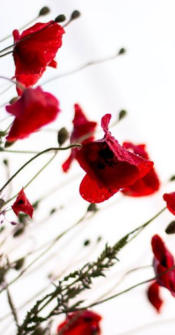5
F or Fr eedom The sky is black ed b y c anno n s mo k e, the smell of c or dit e fill s t h e a ir . The soldier s duck as shells speed b y , s till mo ving f o r w a r d, no time t o s t ar e. Their f allen c o m r a de s l e f t b e hi n d , half buried i n m u d and mir e. The plain tiv e whine of ho r s es as the y tr y t o fr ee the wir e. Then suddenly the sun br eak s thr ough, P eace has c ome a t las t, and t he Killing  fields of No-Man's-Land, no w filled with P o p p ie s R e d, In Memor y of the f al le n who g a v e their all and bled.
S Johnson
N e w s o f M e m b e r s a n d F r i e n d s F r i e n d s w i l l b e s a d t o l e a r n o f t h e d e a t h o f C y n t h i a H a v e l l . C y n t h i a w a s 9 8 y e a r s o l d . S h e c a m e i n t o m e m b e r s h i p a t S t J o h n ' s i n 1 9 3 9 a n d w a s a v e r y a c t i v e m e m b e r o f t h e c h u r c h . S h e s e r v e d a s P r e s i d e n t o f t h e W o m e n ' s F e l l o w s h i p f o r s e v e r a l y e a r s a n d w i l l b e r e m e m b e r e d b y m a n y f o r h e r a c t i v e r o l e i n t h e C h u r c h . A d r i a n
T h o u g h t f o r t h e D a y T o l i v e i n h e a r t s w e l e a v e b e h i n d I s n o t t o d i e . ( F r o m H a l l o w e d G r o u n d b y T h o m a s C a m p b e l l )
St John’s United Reformed C h urch, Ipswich. Nomination of Elders 2020. Nominee’s Name…… ………………… ………………… ………………. I confi rm t h at I have spoken to the above candi date w h o is willing to s tan d for election. Signed: Proposer……… ………………… ………………… …………......... .. . Seconder…… ………………… ………………… ………………… …… Please return this fo rm t o Adrian Cubitt (secretary) By Sunday 15th Novem b e r 2020 (or con t a ctsecretary@stjohnsurcipswich)
Reform magazine 2021 Time to start thinking about next year! If you currently subscribe to Reform (the magazine of the United Reformed Church), I will shortly be putting in an order for the paper copies.  The cost for 2021 is £29.50 for 10 editions usually issued monthly.  I need to know if you want me to order a paper copy. As I’m currently not able to attend Church in person, can you contact me direct so I can arrange this for you.  I would also encourage anyone interested in receiving Reform to contact me.  The digital copy is also available for £17.99, but I suggest you contact Reform direct to arrange this, if this is how you wish to receive the magazine. Andrew Walker   2021 Prayer Handbook and URC diaries   Christmas must be coming! Time to decide if you want to order either the Prayer Handbook or a diary for 2021.  The Prayer Handbook is £4.99 + p&p and the diary is £6.99 +p&p.  The diary is available in either teal or purple, so let me know your colour choice. As I’m currently not able to attend Church in person, can you contact me direct so I can arrange this for you.  Andrew Walker
1 / 1100%